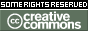REPOZYTORIUM UNIWERSYTETU
W BIAŁYMSTOKUProszę używać tego identyfikatora do cytowań lub wstaw link do tej pozycji: `http://hdl.handle.net/11320/6551`
 Tytuł: Introduction to Stopping Time in Stochastic Finance Theory. Part II Autorzy: Jaeger, Peter Słowa kluczowe: stopping timestochastic process Data wydania: 2017 Data dodania: 11-maj-2018 Wydawca: DeGruyter Open Źródło: Formalized Mathematics, Volume 25, Issue 4, Pages 261–268 Abstrakt: We start proceeding with the stopping time theory in discrete time with the help of the Mizar system , . We prove, that the expression for two stopping times k1 and k2 not always implies a stopping time (k1 + k2) (see Theorem 6 in this paper). If you want to get a stopping time, you have to cut the function e.g. (k1 + k2) ⋂ T (see [2, p. 283 Remark 6.14]). Next we introduce the stopping time in continuous time. We are focused on the intervals [0, r] where r ∈ ℝ. We prove, that for I = [0, r] or I = [0,+∞[ the set {A ⋂ I : A ∈ Borel-Sets} is a σ-algebra of I (see Definition 6 in this paper, and more general given in [3, p.12 1.8e]). The interval I can be considered as a timeline from now to some point in the future. This set is necessary to define our next lemma. We prove the existence of the σ-algebra of the τ -past, where τ is a stopping time (see Definition 11 in this paper and [6, p.187, Definition 9.19]). If τ1 and τ2 are stopping times with τ1 is smaller or equal than τ2 we can prove, that the σ-algebra of the τ1-past is a subset of the σ-algebra of the τ2-past (see Theorem 9 in this paper and [6, p.187 Lemma 9.21]). Suppose, that you want to use Lemma 9.21 with some events, that never occur, see as a comparison the paper  and the example for ST(1)={+∞} in the Summary. We don’t have the element +1 in our above-mentioned time intervals [0, r[ and [0,+1[. This is only possible if we construct a new σ-algebra on ℝ {−∞,+∞}. This construction is similar to the Borel-Sets and we call this σ-algebra extended Borel sets (see Definition 13 in this paper and [3, p. 21]). It can be proved, that {+∞} is an Element of extended Borel sets (see Theorem 21 in this paper). Now we use the interval [0,+∞] as a basis. We construct a σ-algebra on [0,+∞] similar to the book ([3, p. 12 18e]), see Definition 18 in this paper, and call it extended Borel subsets. We prove for stopping times with this given σ-algebra, that for τ1 and τ2 are stopping times with τ1 is smaller or equal than τ2 we have the σ-algebra of the τ1-past is a subset of the σ-algebra of the τ2-past, see Theorem 25 in this paper. It is obvious, that {+∞} 2 extended Borel subsets. In general, Lemma 9.21 is important for the proof of the Optional Sampling Theorem, see 10.11 Proof of (i) in [6, p. 203]. Afiliacja: Siegmund-Schacky-Str. 18a, 80993 Munich, Germany URI: http://hdl.handle.net/11320/6551 DOI: 10.1515/forma-2017-0025 ISSN: 1426-2630 e-ISSN: 1898-9934 Typ Dokumentu: Article Występuje w kolekcji(ach): Formalized Mathematics, 2017, Volume 25, Issue 4

Pliki w tej pozycji:
Plik Opis RozmiarFormat
Pozycja ta dostępna jest na podstawie licencji Licencja Creative Commons CCL